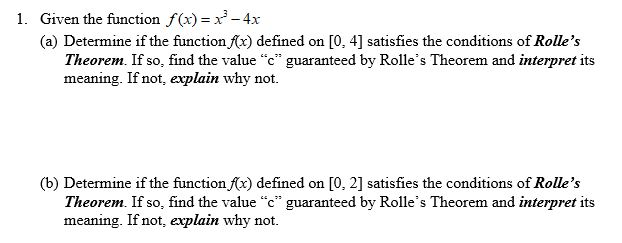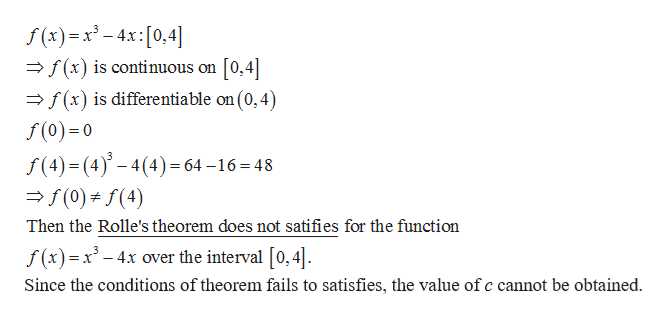# 1. Given the function f(x) x-4x(a) Determine if the function x) defined onTheorem. If so, find the value "c" guaranteed by Rolle's Theorem and interpret itsmeaning. If not, explain why not.[0, 4] satisfies the conditions of Rolle's(b) Determine if the function fx) defined onTheorem. If so, find the value "c" guaranteed by Rolle's Theorem and interpret itsmeaning. If not, explain why not.[0, 2] satisfies the conditions of Rolle's

Question
8 viewshelp_outlineImage Transcriptionclose1. Given the function f(x) x-4x (a) Determine if the function x) defined on Theorem. If so, find the value "c" guaranteed by Rolle's Theorem and interpret its meaning. If not, explain why not. [0, 4] satisfies the conditions of Rolle's (b) Determine if the function fx) defined on Theorem. If so, find the value "c" guaranteed by Rolle's Theorem and interpret its meaning. If not, explain why not. [0, 2] satisfies the conditions of Rolle's fullscreen
check_circle

Step 1

a) To check whether the given function satifies the Rolle\\\\\\'s theorem over the interval [0, 4] or not.help_outlineImage Transcriptionclosef (x)x-4x:[0,4] [0,4 f(x) is continuous on (x) is differentiable on (0,4) f(0) 0 f(4)(4)4(4)64-16 48 (0) (4) Then the Rolle's theorem does not satifies for the function f (x)x-4x over the interval [0,4] Since the conditions of theorem fails to satisfies, the value of c cannot be obtained. fullscreen
Step 2

b) To check whether the given function satifies the Rol...help_outlineImage Transcriptionclosef (x)4x 0,2 -/(x) is Continuous on [0,2 f(x) is differentiable on (0,2) f()0 f(2) (2)4(2) 8-8 0 (0) f(2) Then the Rolle's theorem satifies for the function f(x) x3-4x over the interval [0,2] fullscreen

### Want to see the full answer?

See Solution

#### Want to see this answer and more?

Solutions are written by subject experts who are available 24/7. Questions are typically answered within 1 hour.*

See Solution
*Response times may vary by subject and question.
Tagged in

### Derivative